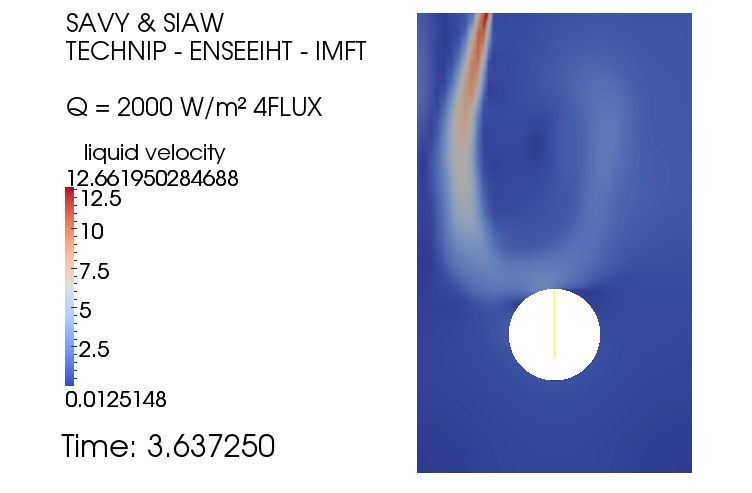# Discussion

The simulations were launched at the first place with the 3 flux model for several values of heat flux desity [Q=2000, 3500, 5000, 10000, 20000 30000] W/m2. The simulations had been ran to represent the boiling process for 5s. Nonetheless, there were errors in the simulations which were found to be caused by high gas volume fraction due to an important heat flux density, imposed as wall boundary condition in static propane. This is because the liquid phase cannot dissipate the heat fast enough, and some small peaks of wall temperature appeared when the cells around the wall are filled with vapour.

A progressive heat flux density was, then, implemented, using the USCLIM.F, so as to create movement in the flow with natural convection (renew the liquid around the tube) that helps to evacuate gas phase. This could prevent the phenomena where the cells around the tube become saturated with gas phase. However, this was found to work for a limited heat flux density up to 6000 W/m².

Animation 1: Evolution of gas volume fraction for a heat flux Q=3000W/m2

Animation 2: Evolution of gas volume fraction for a heat flux Q=5000W/m2

When 3-flux model is used, it is considered that the liquid on the wall, to be used for evaporation, is sufficient to remove the heat from the tube. Superheating of vapour phase is not modelled, which may be the responsible for the errors in the cases with higher heat flux density (high gas void fraction). EDF is developing 4 flux model that models the heat flux absorbed by the steam phase (for more information, please refer to section ' NEPTUNE_CFD - Nucleate Boiling'). Hence, 4 flux model is designed to be more 'robust' than 3 flux as demonstrated in the study performed by Pérez Mañes et al, 2014 , in which 4 flux model was used for a heat flux close to critical heat flux with a gas volume fraction that can reach up to 80 % in the near wall region. Below are displayed some simulations launched with a heat flux density of [5000 6000 10000] W/m².

Animation 3: Evolution of gas volume fraction for a heat flux Q=5000W/m2 with 4 flux model

Animation 4: Evolution of gas volume fraction for a heat flux Q=6000W/m2 with 4 flux model

Animation 5: Evolution of gas volume fraction for a heat flux Q=10000W/m2 with 4 flux model

By comparing the simulations for 5000 W/m², the two models are in good agreement for the instance when the boiling started to take place and also gas volume fraction was produced at the bottom of cylinder, then the bubble plume started to glide upward and activated the other nucleate cavity sites. However, the magnitude of gas volume fraction estimated by 4 flux was higher than 3 flux model. On the other hand, by using 4 flux model, it does not seem to resolve the numerical error that was provoked in the previous discussion. Moreover, it is observed, with listing file, that the error occurred around 6000 W/m² which was the same heat flux density for 3 flux model.

Furthermore, the results obtained, with 3 flux model and 4 flux model, were not very satisfying as the average Tw obtained from NEPTUNE_CFD were overestimated, compare to the experimental result proposed in the literature, due to the presence of stagnation points on the tube. The \$T_w\$ at the stagnation points could be several hundred Kelvin [K] above the saturation temperature and as a consequence, the average \$T_w\$ obtained by the integration around the tube is not comparable with the experimental results.Figure 20: Wall temperature for a heat flux Q=20000W/m2

In addition, it was observed that the flow could be turbulent as shown in the velocity map below, Figure 21. However, backflow was observed throughout the simulation (when heated with 2000W/m²) which might be the major cause of turbulent flow (backflow velocity magnitude could reach approximately 12 m/s) and also it might be the agent that prevented the gas to quit the studied domain, thus paralysed the cooling mechanisms. This backflow was caused by the pressure boundary condition at outlet, as the pressure gradient is fixed at zero. Hence, a depression was produced when the gas volume fraction increased in the studied domain.Figure 21: Velocity map for a heat flux Q=2000W/m2 .Evidence of backflow